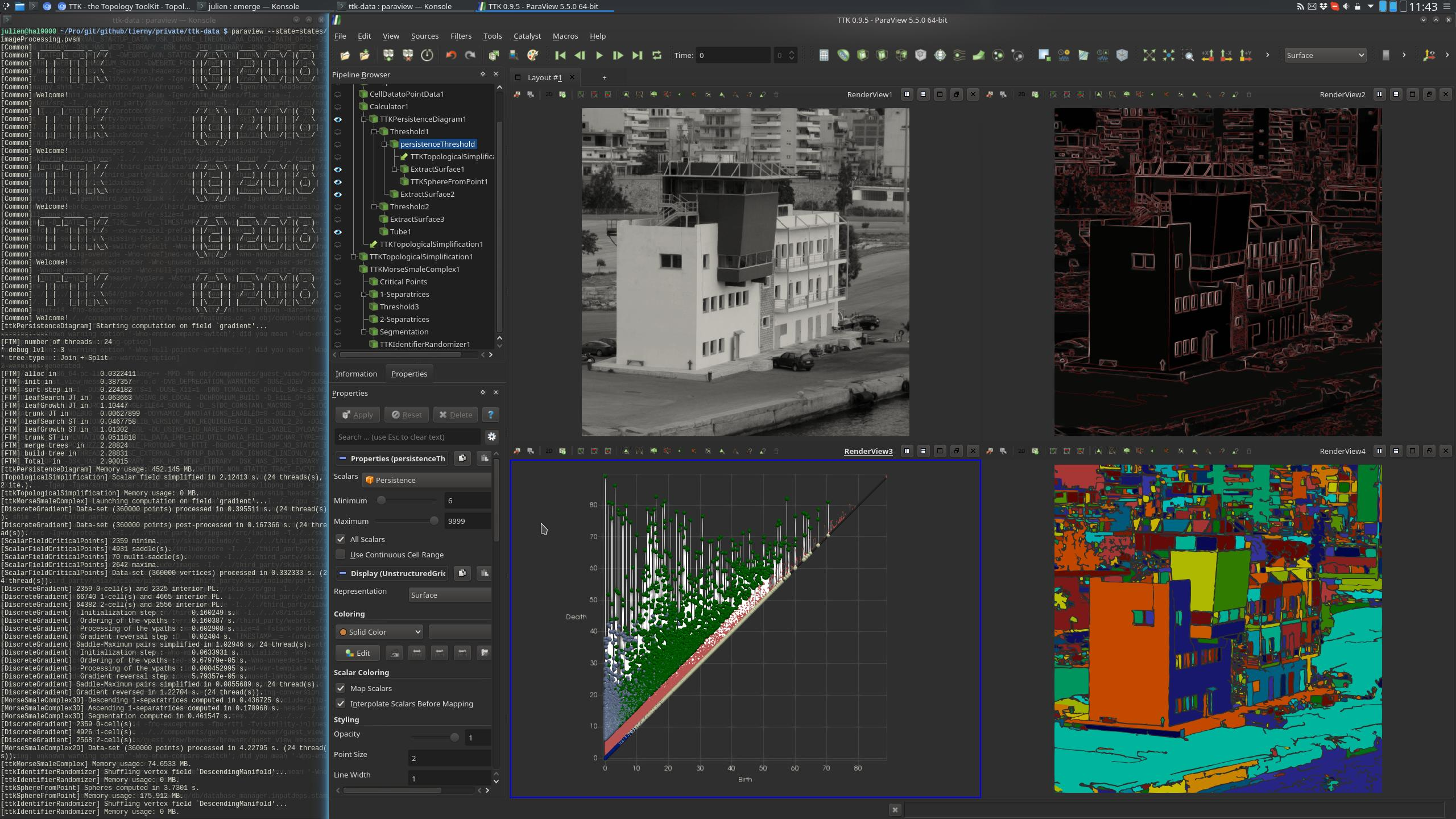# Image Processing¶## Pipeline description¶

This example processes a grayscale image (top left view on the above screenshot) to generate a segmentation. We will construct the segmentation from the image gradient.

First, the image is loaded from disk. The gradient is computed with ParaView's `ComputeDerivatives` or `Gradient` filters. Since TTK only works on scalar field, a `Calculator` is used to compute the gradient magnitude.

From the gradient magnitude, a simplification step involving PersistenceDiagram (bottom left view) and TopologicalSimplification helps removing the noise in the gradient (top right view).

To segment the image, we use the MorseSmaleComplex filter. Since in the input image the objects correspond to low values in the gradient and their edges to high values, we are interested in the `DescendingManifold` scalar field of the `Segmentation` output, whose cells represent regions of low scalar field values.

The IdentifierRandomizer filter is eventually used in order to color neighbor cells with a distinct color (bottom right view on the above screenshot).

## ParaView¶

To reproduce the above screenshot, go to your ttk-data directory and enter the following command:

``````paraview states/imageProcessing.pvsm
``````

## Python code¶

 ``` 1 2 3 4 5 6 7 8 9 10 11 12 13 14 15 16 17 18 19 20 21 22 23 24 25 26 27 28 29 30 31 32 33 34 35 36 37 38 39 40 41 42 43 44 45 46 47 48 49 50 51 52 53 54 55 56 57 58 59 60 61``` ``````#!/usr/bin/env python from paraview.simple import * naturalImagepng = PNGSeriesReader(FileNames=["naturalImage.png"]) # create a new 'Compute Derivatives' computeDerivatives1 = ComputeDerivatives(Input=naturalImagepng) computeDerivatives1.Scalars = ["POINTS", "PNGImage"] # create a new 'Cell Data to Point Data' cellDatatoPointData1 = CellDatatoPointData(Input=computeDerivatives1) # create a new 'Calculator' calculator1 = Calculator(Input=cellDatatoPointData1) calculator1.ResultArrayName = "gradient" calculator1.Function = "mag(ScalarGradient)" # create a new 'TTK PersistenceDiagram' tTKPersistenceDiagram1 = TTKPersistenceDiagram(Input=calculator1) tTKPersistenceDiagram1.ScalarField = ["POINTS", "gradient"] # create a new 'Threshold' threshold1 = Threshold(Input=tTKPersistenceDiagram1) threshold1.Scalars = ["CELLS", "PairIdentifier"] threshold1.ThresholdMethod = "Between" threshold1.LowerThreshold = 0.0 threshold1.UpperThreshold = 999999999 # create a new 'Threshold' persistenceThreshold = Threshold(Input=threshold1) persistenceThreshold.Scalars = ["CELLS", "Persistence"] persistenceThreshold.ThresholdMethod = "Between" persistenceThreshold.LowerThreshold = 6.0 persistenceThreshold.UpperThreshold = 999999999 # create a new 'TTK TopologicalSimplification' tTKTopologicalSimplification1 = TTKTopologicalSimplification( Domain=calculator1, Constraints=persistenceThreshold, ) tTKTopologicalSimplification1.ScalarField = ["POINTS", "gradient"] # create a new 'TTK MorseSmaleComplex' tTKMorseSmaleComplex1 = TTKMorseSmaleComplex(Input=tTKTopologicalSimplification1) tTKMorseSmaleComplex1.ScalarField = ["POINTS", "gradient"] # create a new 'Threshold' threshold3 = Threshold(Input=OutputPort(tTKMorseSmaleComplex1, 1)) threshold3.Scalars = ["CELLS", "SeparatrixType"] threshold3.ThresholdMethod = "Between" threshold3.LowerThreshold = 1.0 threshold3.UpperThreshold = 1.0 # create a new 'TTK IdentifierRandomizer' tTKIdentifierRandomizer1 = TTKIdentifierRandomizer( Input=OutputPort(tTKMorseSmaleComplex1, 3), ) tTKIdentifierRandomizer1.ScalarField = ["POINTS", "DescendingManifold"] SaveData("Segmentation.vti", tTKIdentifierRandomizer1) ``````

To run the above Python script, go to your ttk-data directory and enter the following command:

``````pvpython python/imageProcessing.py
``````

## Outputs¶

• `Segmentation.vti`: the image segmentation output.

## C++/Python API¶

IdentifierRandomizer

MorseSmaleComplex

PersistenceDiagram

TopologicalSimplification